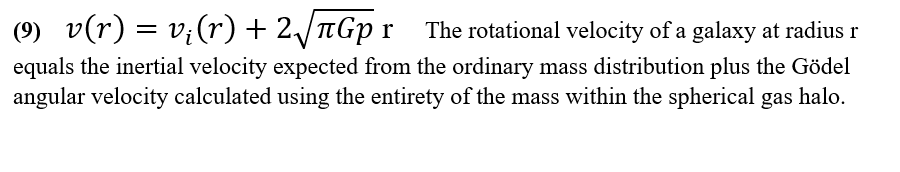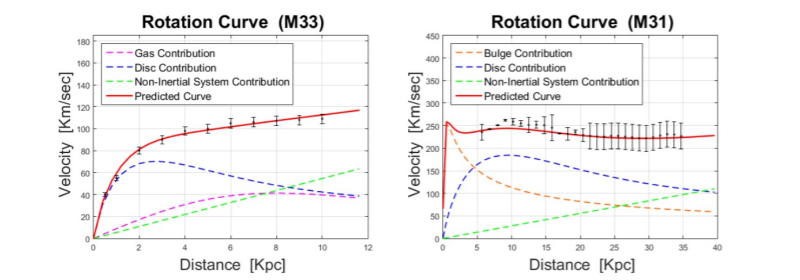Monday, 22 February 2021 17:02

Equation 9Equation 9

Galaxies rotate but they do not rotate in accordance with our standard ideas about gravity.

A large discrepancy exists between the rotation curves for disc galaxies expected from a classical or relativistic consideration of their baryonic mass distributions, and the rotation curves observed. This discrepancy has led to the hypothesis that a mysterious ‘Dark Matter’ must make up the difference.

Gomel and Zimmerman show that an apparently ‘Non -Inertial System Component’ in the form of an angular velocity ‘w’, can account for this discrepancy.

v(r) = vi(r) + w(r)This paper asserts that the angular velocity w arises from a Gödelian rotation component of the galaxies where w = 2sqrt (pi G p)

‘Matter everywhere rotates with an angular velocity of twice the square root of pi times the gravitational constant times the density.’

Thus, v(r) = vi(r) = 2sqrt(pi G p)  r

Now because the wr component of galactic rotation dominates at the extremities of galactic discs, thus creating ‘flat’ rotation curves, and because the outer edges of all disc galaxies rotate with the same time period of almost exactly one billion years, irrespective of their varied sizes.

It follows that we can calculate w with high accuracy to w = 2 e-16 radians/second.

Calculation: distance/velocity = time

2 pi r / wr = t

2 pi / 2 e-16 = t

pi e16 = t = 3.142 e16 seconds. (A billion years equals 3.155 e16 seconds)

Thus, it seems far more likely that all disc galaxies have a Gödelian rotational component of precisely 2 e-16 radians/second rather than dark matter halos with sizes and masses precisely and mysteriously tailored for each galaxy’s distribution of ordinary baryonic matter to create the observed rotation curves.

(Aside - just a little aside I came across whilst reading science news daily site - a flurry of papers have come out about 'Self-Scattering Dark Matter', apparently it will have to have this amongst its other miraculous properties to account for some of its predicted distribution. Phlogiston ended up with a negative weight variety.)